##### Pre-Calculus For Dummies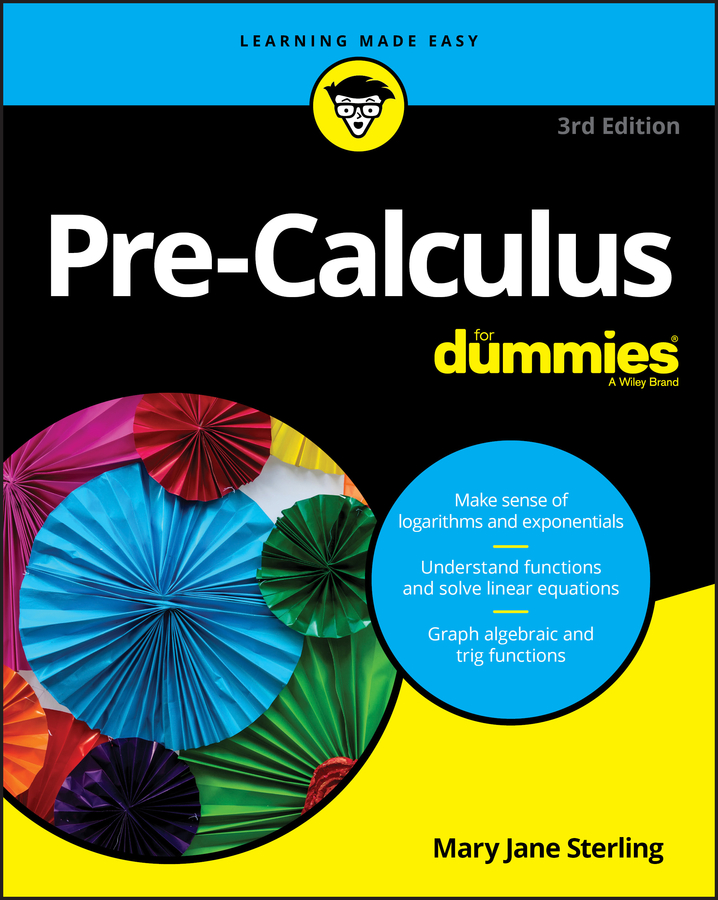Three trigonometric ratios — secant, cosecant, and cotangent — are called reciprocal functions because they're the reciprocals of sine, cosine, and tangent. These three functions open up three more ways in which you can solve equations in pre-calculus. The following list breaks down these functions and how you use them:

• Cosecant, or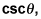is the reciprocal of sine. The reciprocal of a number a is 1/a, so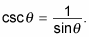Because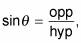you see that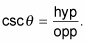In other words, cosecant is the ratio of the hypotenuse to the opposite leg.

• Secant, or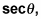is the reciprocal of cosine: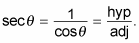Secant, in other words, is the ratio of the hypotenuse to the adjacent leg.

A common mistake is to think that secant is the reciprocal of sine and that cosecant is the reciprocal of cosine, but the previous bullets illustrate the truth.

• Cotangent, or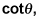is the reciprocal of tangent. (How's that for obvious?) You have the hang of this if you've looked at the previous bullets: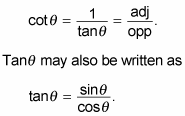Therefore,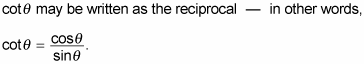Remember that secant, cosecant, and cotangent are all reciprocals, but you typically won't find a button for them on your calculator. You must use their reciprocals — sine, cosine, and tangent. Don't get confused and use the sin–1, cos–1, and tan–1 buttons, either. Those buttons are for the inverse trig functions.